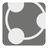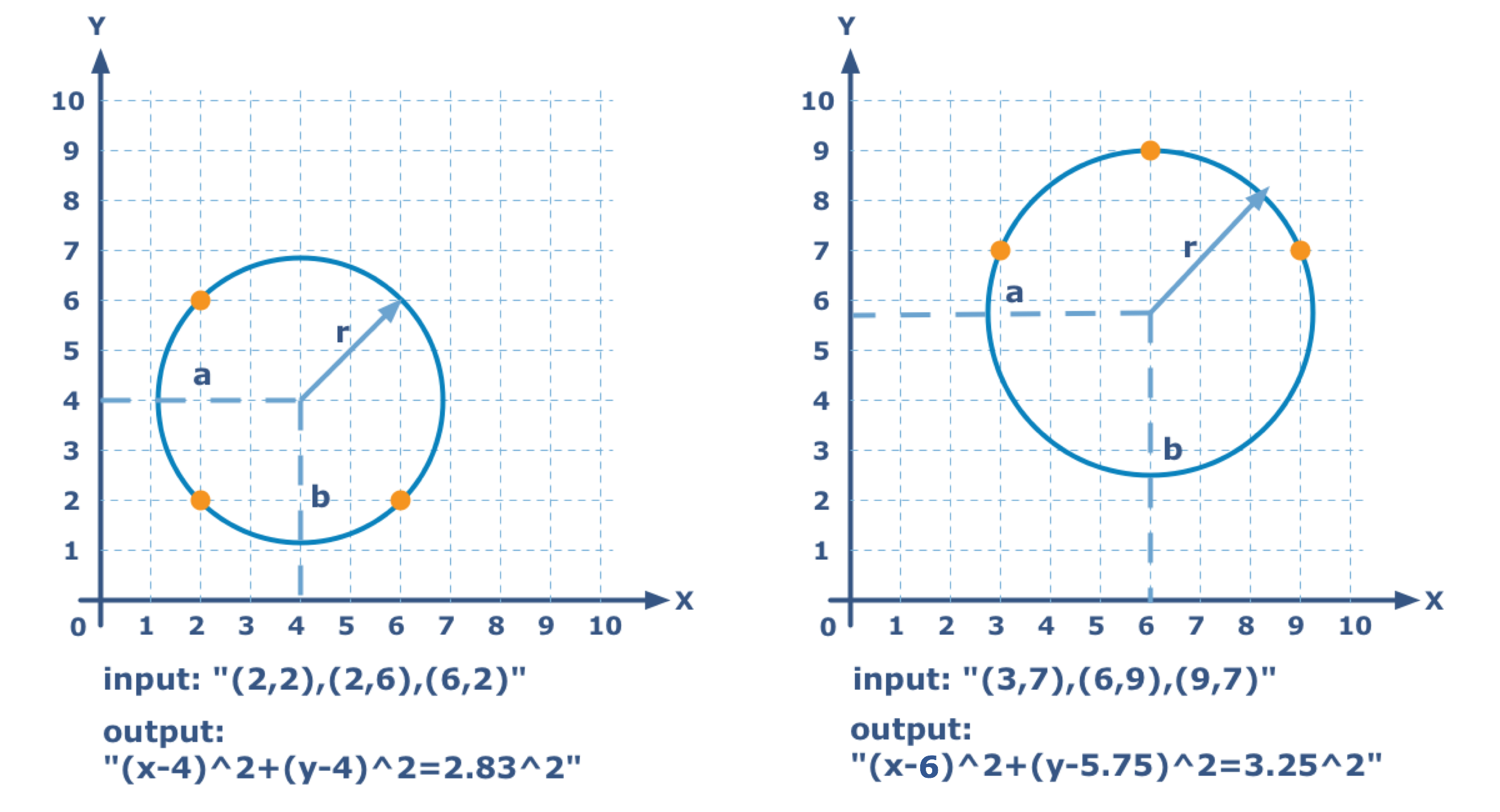# Three Points Circle

Simple
English FR RU

Nicola discovered a caliper inside a set of drafting tools he received as a gift. Seeing the caliper, he has decided to learn how to use it.

Through any three points that do not exist on the same line, there lies a unique circle. The points of this circle are represented in a string with the coordinates like so:

```    "(x1,y1),(x2,y2),(x3,y3)"

```

Where x1,y1,x2,y2,x3,y3 are digits.

You should find the circle for three given points, such that the circle lies through these point and return the result as a string with the equation of the circle. In a Cartesian coordinate system (with an X and Y axis), the circle with central coordinates of (x0,y0) and radius of r can be described with the following equation:

```    "(x-x0)^2+(y-y0)^2=r^2"

```

where x0 , y0 , r are decimal numbers rounded to two decimal points . Remove extraneous zeros and all decimal points, they are not necessary. For rounding, use the standard mathematical rules.Input: Coordinates as a string..

Output: The equation of the circle as a string.

Example:

```checkio("(2,2),(6,2),(2,6)") == "(x-4)^2+(y-4)^2=2.83^2"
checkio("(3,7),(6,9),(9,7)") == "(x-6)^2+(y-5.75)^2=3.25^2"```

How it is used: This equation, also known as Equation of the Circle, comes from the Pythagorean theorem when applied to any point on a circle: the radius is the hypotenuse of a right-angled triangle whose other sides are of length x − a and y − b. Of course you can use this concept for you mathematics software, but we just want to remind about how awesome circles are.

Precondition: All three given points do not lie on one line.
0 < x i , y i , r < 1045
Settings
Code:
Other:
Invalid hot key. Each hot key should be unique and valid
Hot keys:
•  to Run Code: to Check Solution: to Stop:
CheckiO Extensions

CheckiO Extensions allow you to use local files to solve missions. More info in a blog post.

In order to install CheckiO client you'll need installed Python (version at least 3.8)

Install CheckiO Client first:

`pip3 install checkio_client`

`checkio --domain=py config --key=`

Sync solutions into your local folder

`checkio sync`

(in beta testing) Launch local server so your browser can use it and sync solution between local file end extension on the fly. (doesn't work for safari)

`checkio serv -d`

Alternatevly, you can install Chrome extension or FF addon

`checkio install-plugin`
`checkio install-plugin --ff`
`checkio install-plugin --chromium`

Read more here about other functionality that the checkio client provides. Feel free to submit an issue in case of any difficulties.